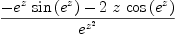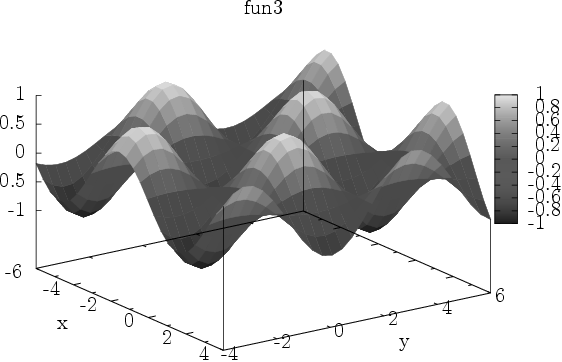login  home  contents  what's new  discussion  bug reports help  links  subscribe  changes  refresh  edit

Demonstrate how graphs may be plotted and displayed from MathAction

By: David Cyganski, Tue, Jul 24, 2007 at 8:16 AM.

I have written an External Method that can be called from a MathAction web page to generate graphics that can be displayed on the page that created them. The "gnupyplot" External Method uses a python script to call gnuplot to generate 2D and 3D graphics. The link between MathAction and gnupyplot is the same LocalFS "images" filesystem used by MathAction which is found in the var/LatexWiki subdirectory of the zope instance.

The demonstration below is still "unpackaged" and needs to be pulled into the form of a few more flexible functions, but the utility is fairly obvious at this stage. This packaging would involve setting it up to pass one or more functions in a single call for plotting, choosing colors for each, which can be done with some careful "map" crafting and function overloading.

The "gnuplot" function samples a given expression over a given range of values and writes the results into a file (with the name testfile2.dat in the following example). We will have to use DoubleFloats (for speed) expressed as text floats (for compatibility with gnuplot). The format and method of getting there is the result of a great deal of hacking and experimenting due to many non-obvious limitations, defaults and assorted odd behaviors on Axiom's part when it comes to the treatment of doubles as strings and the saving of text to text files!

The expression argument for gnuplot can be any expression in any single variable. The variable name is declared as part of the range argument which takes a form such as x=0..50 if we wish to plot some expression in x over 0 to 50.

fricas
gnuplot(f,segbind,filename)==
--Define number of function points to evaluate
xpoints:=300
xlo:=lo(segment(segbind))
xhi:=hi(segment(segbind))
xrange:DoubleFloat:=xhi-xlo
xvar:=variable(segbind)
dx:DoubleFloat:=xrange/(xpoints-1)
--outputFloating(5)
--outputSpacing(0)
f1:TextFile:=open(filename,"output")
for xindex in 0..(xpoints-1) repeat
writeLine!(f1,concat( [unparse((xindex*dx)::InputForm)," ", _
unparse(eval(f, xvar=xindex*dx+xlo)::InputForm)]) )
close! f1
Type: Void

fricas
gnuplot(sin(z),z=0..30,"testfile2.dat")
There are no library operations named lo
Use HyperDoc Browse or issue
)what op lo
to learn if there is any operation containing " lo " in its name.
Cannot find a definition or applicable library operation named lo
with argument type(s)
Segment(NonNegativeInteger)
Perhaps you should use "@" to indicate the required return type,
or "$" to specify which version of the function you need. FriCAS will attempt to step through and interpret the code. There are no library operations named lo Use HyperDoc Browse or issue )what op lo to learn if there is any operation containing " lo " in its name. Cannot find a definition or applicable library operation named lo with argument type(s) Segment(NonNegativeInteger) Perhaps you should use "@" to indicate the required return type, or "$" to specify which version of the function you need.

gnupyplot3.py.txt

On Tue, Jun 24, 2008 at 8:16 PM Bill Page wrote:

Instead of using the original external script, I have installed gnuplot and gnuplottex to permit gnuplot commands to be embedded in wiki pages using:

  \begin{gnuplot}
...
\end{gnuplot}


Here are some good examples of gnuplot scripts.

So now we can plot the data using the commands:

  \begin{gnuplot}[terminal=pslatex,terminaloptions=color,scale=1.3]
set title "sin(z),z=0..30"
plot "testfile2.dat" title "" with lines
\end{gnuplot}


\begin{gnuplot}[terminal=pslatex,terminaloptions=color,scale=1.3]? set title "sin(z),z=0..30" plot "testfile2.dat" title "" with lines \end{gnuplot}

By changing a single statement in the gnupyplot function we can have the image display in an Xwindow, hence one can easily use the same code but for this one parametric change for both interactive and MathAction application.

Of course this can also be done directly using just gnuplot:

  \begin{gnuplot}[terminal=pslatex,terminaloptions=color,scale=1.3]
plot [z=0:30] [-1.0:1.0] sin(z) title "" with lines
\end{gnuplot}


\begin{gnuplot}[terminal=pslatex,terminaloptions=color,scale=1.3]? plot [z=0:30]? [-1.0:1.0]? sin(z) title "" with lines \end{gnuplot}

I would like to add the ability to handle lists of functions by overloading gnuplot to accept the single expression or a list. Simpling writing the additional values for each curve successively onto the same line of the data file will allow gnupyplot to generate such plots. Only a minor change needs to be made to gnupyplot to support this generality.

One could also use a similar approach to directly display MathAction draw function graphs. What we have to do is write a string file output function that reads the format of the "vp" port data generated by Axiom and convert it to the simple textfile, one point per line, style needed by gnuplot.

In order to take advantage of function name overloading it is desirable to write this code in SPAD. That allows us to call gnuDraw in much the same way as we would call draw in Axiom. This routine uses draw internally to create the plot data structure.

)abbrev package GDRAW OldGnuDraw
++ Author: Bill Page and David Cyganski
++ Date: June 25, 2008
++ Description:
++ This package provides support for gnuplot. These routines
++ generate output files contain gnuplot scripts that may be
++ processed directly by gnuplot. This is especially convenient
++ in the axiom-wiki environment where gnuplot is called from
++ LaTeX via gnuplottex.
EF ==> Expression Float
SBF ==> SegmentBinding Float
DROP ==> DrawOption
DROP0 ==> DrawOptionFunctions0
STR ==> String
LDF ==> List DoubleFloat
OldGnuDraw(): with
gnuDraw:(EF, SBF, STR, List DROP)->Void
++ \spad{gnuDraw} provides 2d plotting with options
gnuDraw:(LDF,LDF, STR, List DROP)->Void
++ \spad{gnuDraw} provides 2d plotting from list of values with options
gnuDraw:(EF, SBF, STR)->Void
++ \spad{gnuDraw} provides 2d plotting, default options
gnuDraw:(LDF,LDF, STR)->Void
++ \spad{gnuDraw} provides 2d plotting from list of values, default options
gnuDraw:(EF, SBF, SBF, STR, List DROP)->Void
++ \spad{gnuDraw} provides 3d surface plotting with options
gnuDraw:(EF, SBF, SBF, STR)->Void
++ \spad{gnuDraw} provides 3d surface plotting, default options
-- 2-d plotting
gnuDraw(f:EF,segbind:SBF,filename:STR,opts:List DROP):Void ==
import TwoDimensionalViewport, GraphImage, TopLevelDrawFunctions EF
f1:TextFile:=open(filename::FileName,"output")
-- handle optional parameters
writeLine!(f1,concat(["set title _"",title(opts,"")$DROP0,"_""])) writeLine!(f1,"plot '-' title '' lw 3 with lines") -- extract data as List List Point DoubleFloat p2:=pointLists(getGraph(draw(f, segbind),1)); for p1 in p2 repeat for p in p1 repeat writeLine!(f1,concat([unparse(convert(p.1)@InputForm)," ", unparse(convert(p.2)@InputForm)])) writeLine!(f1); -- blank line need to mark a "branch" close! f1 gnuDraw(l1:LDF,l2:LDF,filename:STR,opts:List DROP):Void == import TwoDimensionalViewport, GraphImage, TopLevelDrawFunctionsForPoints f1:TextFile:=open(filename::FileName,"output") -- handle optional parameters writeLine!(f1,concat(["set title _"",title(opts,"")$DROP0,"_""]))
writeLine!(f1,"plot '-' title '' lw 3 with lines")
-- extract data as List List Point DoubleFloat
p2:=pointLists(getGraph(draw(l1, l2),1));
for p1 in p2 repeat
for p in p1 repeat
writeLine!(f1,concat([unparse(convert(p.1)@InputForm)," ",
unparse(convert(p.2)@InputForm)]))
writeLine!(f1); -- blank line need to mark a "branch"
close! f1
-- default title is ""
gnuDraw(f:EF,segbind:SBF,filename:STR):Void ==
gnuDraw(f,segbind,filename,[title("")$DROP]) gnuDraw(l1:LDF,l2:LDF,filename:STR):Void == gnuDraw(l1,l2,filename,[title("")$DROP])
-- 3-d plotting
gnuDraw(f:EF,segbind1:SBF, segbind2:SBF, filename:STR, opts:List DROP):Void ==
import SubSpace, ThreeSpace DoubleFloat, TopLevelDrawFunctions EF
f1:TextFile:=open(filename::FileName,"output")
-- process optional parameters
writeLine!(f1,concat(["set title _"",title(opts,"")$DROP0,"_""])) writeLine!(f1,"splot '-' title '' with pm3d") -- extract data as List List Point DoubleFloat p2:=mesh(subspace(draw(f, segbind1, segbind2))); for p1 in p2 repeat for p in p1 repeat writeLine!(f1,concat([unparse(convert(p.1)@InputForm)," ", unparse(convert(p.2)@InputForm)," ", unparse(convert(p.3)@InputForm)])) writeLine!(f1); -- blank line need to mark a "branch" close! f1 -- default title is "" gnuDraw(f:EF,segbind1:SBF, segbind2:SBF, filename:STR):Void == gnuDraw(f,segbind1,segbind2,filename,[title("")$DROP])
   Compiling FriCAS source code from file
using old system compiler.
GDRAW abbreviates package OldGnuDraw
------------------------------------------------------------------------
initializing NRLIB GDRAW for OldGnuDraw
compiling into NRLIB GDRAW
compiling exported gnuDraw : (Expression Float,SegmentBinding Float,String,List DrawOption) -> Void
Time: 0.38 SEC.
compiling exported gnuDraw : (List DoubleFloat,List DoubleFloat,String,List DrawOption) -> Void
Time: 0.04 SEC.
compiling exported gnuDraw : (Expression Float,SegmentBinding Float,String) -> Void
Time: 0 SEC.
compiling exported gnuDraw : (List DoubleFloat,List DoubleFloat,String) -> Void
Time: 0 SEC.
compiling exported gnuDraw : (Expression Float,SegmentBinding Float,SegmentBinding Float,String,List DrawOption) -> Void
Time: 0.26 SEC.
compiling exported gnuDraw : (Expression Float,SegmentBinding Float,SegmentBinding Float,String) -> Void
Time: 0.01 SEC.
(time taken in buildFunctor:  0)
;;;     ***       |OldGnuDraw| REDEFINED
;;;     ***       |OldGnuDraw| REDEFINED
Time: 0 SEC.
Cumulative Statistics for Constructor OldGnuDraw
Time: 0.69 seconds
finalizing NRLIB GDRAW
Processing OldGnuDraw for Browser database:
--------constructor---------
--------(gnuDraw ((Void) (Expression (Float)) (SegmentBinding (Float)) (String) (List (DrawOption))))---------
--------(gnuDraw ((Void) (List (DoubleFloat)) (List (DoubleFloat)) (String) (List (DrawOption))))---------
--------(gnuDraw ((Void) (Expression (Float)) (SegmentBinding (Float)) (String)))---------
--------(gnuDraw ((Void) (List (DoubleFloat)) (List (DoubleFloat)) (String)))---------
--------(gnuDraw ((Void) (Expression (Float)) (SegmentBinding (Float)) (SegmentBinding (Float)) (String) (List (DrawOption))))---------
--------(gnuDraw ((Void) (Expression (Float)) (SegmentBinding (Float)) (SegmentBinding (Float)) (String)))---------
; compiling file "/var/aw/var/LatexWiki/GDRAW.NRLIB/GDRAW.lsp" (written 04 APR 2022 07:05:56 PM):
; /var/aw/var/LatexWiki/GDRAW.NRLIB/GDRAW.fasl written
; compilation finished in 0:00:00.048
------------------------------------------------------------------------
OldGnuDraw is now explicitly exposed in frame initial
OldGnuDraw will be automatically loaded when needed from
/var/aw/var/LatexWiki/GDRAW.NRLIB/GDRAW

fricas
D(cos(exp(z))/exp(z^2),z)(1)
Type: Expression(Integer)
fricas
gnuDraw(%,z=-5..5,"testfile1.dat",title=="fun2")
fricas
Compiling function %C with type DoubleFloat -> DoubleFloat
Type: Void

fricas
gnuDraw(sin(x)*cos(y),x=-6..4,y=-4..6,"testfile4.dat",title=="fun3")
fricas
Compiling function %E with type (DoubleFloat, DoubleFloat) ->
DoubleFloat
Type: Void

When I tried to compile OldGnuDraw in FriCAS 1.0.9 I found that this line produced an error

import SubSpace?, ThreeSpace? DoubleFloat?, TopLevelDrawFunctions? EF

It worked when I changed it to:

import SubSpace?(3,DoubleFloat?), ThreeSpace? DoubleFloat?, TopLevelDrawFunctions? EF

otherwise I am finding this code very useful, thanks, Martin BakerSubject: (replying)   Be Bold !! ( 14 subscribers )